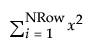Publication date: 11/10/2021

## Summation

The Summation() function adds the body results over all i values. The syntax is:

`Summation( initialization, limitvalue, body );`

For example:

`s = Summation( i = 1, 10, i );`

returns 55, the result of 1+2+3+4+5+6+7+8+9+10.

The script works like this:

 s = Sets the s variable to the value of the function. Summation( Begins the Summation() loop. i = 1, Sets i to 1. 10, Sets the limit of i to 10. i All values of i from 1 to 10 are added together, resulting in 55. ); Ends the loop.

This behavior is similar to Σ in the Formula Editor. The following expression:

`Summation( i = 1, N Row(), x ^ 2 );`

is equivalent to the following formula in the Formula Editor:

``